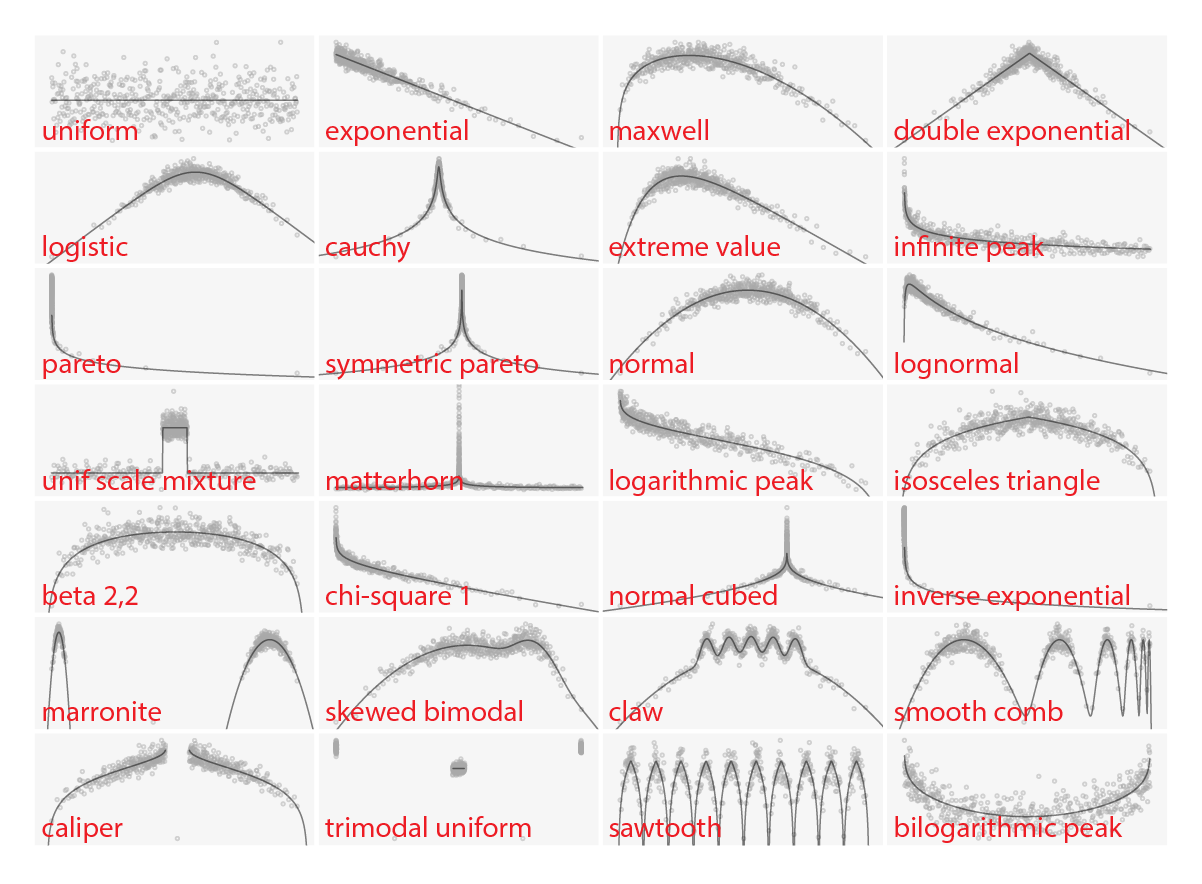# ed

Ed is a nonparametric density estimation method that turns density estimation into a regression problem, providing much-needed diagnostics for evaluating goodness-of-fit and visualization for identifying interesting features without being influenced by artifacts introduced by the estimation method. It also helps deal with some common problems encoutered using traditional KDE approaches, such as fixed bandwidths, boundaries / discontinuities, bias, and choosing optimal smoothing parameters. A preprint of a paper describing the method is available here.

Ed raw estimates of the 28 "benchden" benchmark densities with the true density superposed:## Install:

``````devtools::install_github("hafen/ed")
``````

To recreate the plot above:

``````ed_plot(~ x | density, data = benchdat,
scales = list(relation = "free", draw = FALSE),
panel = function(x, y, ..., subscripts) {
panel.ed(x, ...)
tmp <- ed_raw(x)
ss <- seq(min(tmp\$x), max(tmp\$x), length = 200)
panel.lines(ss, log(benchden::dberdev(ss,
dnum = benchdat\$dennum[subscripts])), col = "black", lwd = 2)
},
cex = 0.3,
between = list(x = 0.25, y = 0.25),
aspect = 1,
layout = c(7, 4),
as.table = TRUE
)
``````

hafen/ed documentation built on May 17, 2019, 1:32 p.m.